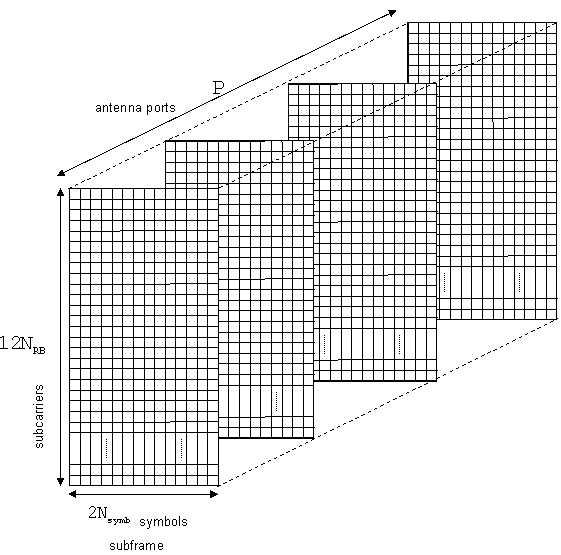## Representing Resource Grids

### Overview

This section describes the data structures used to represent the resource grid in the LTE Toolbox™.

### Multidimensional Arrays

Before OFDM modulation (IFFT), the physical channels and signals in LTE are mapped to different portions of the resource grid. LTE Toolbox represents the resource grid as a multidimensional array.The rows of this array represent the subcarrier. The columns map the OFDM or SC-FDMA symbols in the downlink and uplink, respectively. The third dimension or plane represents the antenna ports. In the LTE Toolbox, the resource grid spans a subframe in the time-domain, instead of a slot. Hence, the documentation uses the term subframe resource grid. The size of this multidimensional array is 12NRB×2Nsymb×P, where NRB is the number of resource blocks spanning the available bandwidth, Nsymb is the number of OFDM, or SC-FDMA in the uplink, symbols per slot, and P is the number of antenna ports. Therefore, the resource grid represents a subframe,two slots, and whole bandwidth since there are 12 subcarriers per resource block. For the single antenna case, you can work with a two-dimensional array of size 12NRB×2Nsymb.

### Creating an Empty Resource Grid

Create an empty downlink resource grid using two different methods. Valid and equivalent subframe resource grids can be created using the `lteDLResourceGrid` function or the MATLAB® `zeros` function.

Initialize required parameters

Create the parameter structure for normal cyclic prefix, nine downlink resource blocks, and one transmit antenna. Also define seven symbols per slot for use in the `zeros` function only.

```enb.CyclicPrefix = 'Normal'; enb.NDLRB = 9; enb.CellRefP = 1; noSymbolsSlot = 7;```

Create two empty resource grids

Create an empty subframe resource grid, using each method.

```resourceGrid1 = lteDLResourceGrid(enb); resourceGrid2 = zeros(enb.NDLRB*12, noSymbolsSlot*2, enb.CellRefP);```

Confirm the grids are equal

Compare the two grid variables for equality using the MATLAB `isequal` function.

`isequal(resourceGrid1,resourceGrid2)`
```ans = logical 1 ```

Both approaches generate the same result. Use either approach to create an empty downlink resource grid. Similarly, an empty uplink resource grid could be created using `lteULResourceGrid` function or the MATLAB `zeros` function.